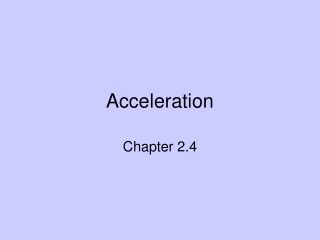DownloadDownload PresentationAcceleration

# Acceleration

Télécharger la présentation## Acceleration

- - - - - - - - - - - - - - - - - - - - - - - - - - - E N D - - - - - - - - - - - - - - - - - - - - - - - - - - -
##### Presentation Transcript

1. Acceleration Chapter 2.4

2. acceleration • Rate at which velocity is changing acceleration= change of velocity time Acceleration = change of speed (along a straight line) time

3. Acceleration occurs when there is either a change in speed, change in direction or both (whenever there is a change in velocity).

4. In physics, acceleration applies to both increases and decreases in speed • Decrease in speed= negative acceleration

5. Practice Problem • Suppose a car moving in a straight line steadily increases its speed each second, first from 35 to 40 km/h, then from 40 to 45 km/h, them from 45 to 50 km/h. What is its acceleration? • Speed increases 5 km/h each 1 s interval, therefore acceleration= 5 km/h*s (read 5 km per hour second)

6. Practice Problem 2 • In 5 seconds a car moving in a straight line increases its speed from 50 km/h to 65 km/h, while a truck goes from rest to 15 km/h in a straight line. Which undergoes the greater acceleration? What is the acceleration of each vehicle? • Acceleration for both vehicles is 15 km/h*s (the undergo the same acceleration even though their speeds are quite different)

7. Speed vs. Time Graphs • X axis: always time • Y axis: always speed • Slope of line = speed/time • Slope tells you what happens to acceleration of the object • Area under line tells you the distance the object traveled.

8. What is happening in this graph? • Objected started at rest • Speed is increasing • Object is accelerating

9. What is happening in this graph? • Object is slowing down • Object is negatively accelerating (decelerating)

10. What is happening in this graph? • Both objects are moving • Both objects are increasing speed but at different rates • Dashed line increases speed faster so it has the greater acceleration

11. What is happening in this graph?